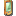# Vector3 Members

Include Protected Members
Include Inherited Members

Include .NET Framework Members

Include .NET Compact Framework Members
Include XNA Framework Members

[This documentation is for preview only, and is subject to change in later releases. Blank topics are included as placeholders.]

Describes and manipulates a vector in three-dimensional (3-D) space.

The Vector3 type exposes the following members.

## Constructors

Name DescriptionVector3 Initializes a new instance of the Vector3 structure.

Top

## Methods

Name DescriptionAdd Overloaded. Adds two 3-D vectors.Cross Determines the cross product of the two specified 3-D vectors.Dot Determines the dot product of the two specified 3-D vectors.Equals Returns a value that indicates whether the current instance is equal to a specified object. (Overrides ValueType.Equals(Object).)Finalize Allows an Object to attempt to free resources and perform other cleanup operations before the Object is reclaimed by garbage collection. (Inherited from Object.)GetHashCode Returns the hash code for the current instance. (Overrides ValueType.GetHashCode().)GetType Gets the Type of the current instance. (Inherited from Object.)Length Overloaded. Returns the length of a 3-D vector.LengthSq Overloaded. Returns the square of the length of a 3-D vector.Lerp Performs a linear interpolation between two 3-D vectors.Maximize Overloaded. Creates a 3-D vector that is made up of the largest components of two 3-D vectors.MemberwiseClone Creates a shallow copy of the current Object. (Inherited from Object.)Minimize Overloaded. Creates a 3-D vector that is made up of the smallest components of two 3-D vectors.Multiply Multiplies a 3-D vector.Normalize Overloaded. Creates a normalized version of a 3-D vector.Project Overloaded. Projects a vector from object space into screen space.Scale Overloaded. Scales a 3-D vector.Subtract Overloaded. Subtracts two 3-D vectors.ToString Returns a String that represents the current Object. (Inherited from Object.)Transform Overloaded. Transforms a specified 3-D vector or array of 3-D vectors using a given matrix.TransformCoordinate Overloaded. Transforms a 3-D vector or an array of 3-D vectors using a given matrix, projecting the result back into w = 1.TransformNormal Overloaded. Transforms a 3-D vector normal using the specified matrix.Unproject Overloaded. Projects a vector from screen space into object space.

Top

## Operators

Name DescriptionAddition Adds two 3-D vectors.Equality Compares two 3-D vectors to determine whether they are the same.Inequality Compares two 3-D vectors to determine whether they are different.Multiply Overloaded. Determines the product of a number and a 3-D vector.Subtraction Subtracts a 3-D vector from another 3-D vector.UnaryNegation Returns a 3-D vector that is the negation of the specified 3-D vector.

Top

## Fields

Name DescriptionX Represents the x component of the 3-D vector.Y Represents the y component of the 3-D vector.Z Represents the z component of the 3-D vector.

Top

## Properties

Name DescriptionEmpty Retrieves an empty 3-D vector.

Top